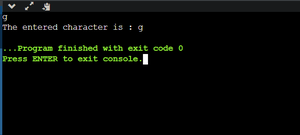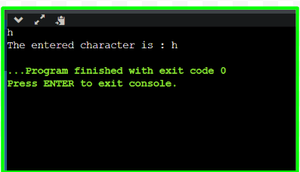GeeksforGeeks App
Open AppBrowser
Continue

# C++ getchar() Function

getchar( ) is a function that takes a single input character from standard input. The major difference between getchar( ) and getc( ) is that getc( ) can take input from any number of input streams but getchar( ) can take input from a single standard input stream.

It is present inside the stdin.h C library. Just like getchar, there is also a function called putchar( ) that prints only one character to the standard output screen

Syntax:

`int getchar(void);`

Return Type: The input from the standard input is read as an unsigned char and then it is typecasted and returned as an integer value(int) or EOF(End Of File). A EOF is returned in two cases, 1. when file end is reached. 2. When there is an error during execution.

Example:

## C++

 `// C++ Program to implement``// Use of getchar()``#include ``#include `` ` `using` `namespace` `std;`` ` `int` `main()``{``    ``char` `x;`` ` `    ``x = ``getchar``();``    ``cout << ``"The entered character is : "` `<< x;`` ` `    ``return` `0;``}`

Output:Output

Example :

## C++

 `// C++ Program to implement``// Use of getchar() and putchar()``#include ``#include `` ` `using` `namespace` `std;`` ` `int` `main()``{``    ``char` `x;`` ` `    ``x = ``getchar``();`` ` `    ``cout << ``"The entered character is : "``;``    ``putchar``(x);`` ` `    ``return` `0;``}`

Output:Output

My Personal Notes arrow_drop_up